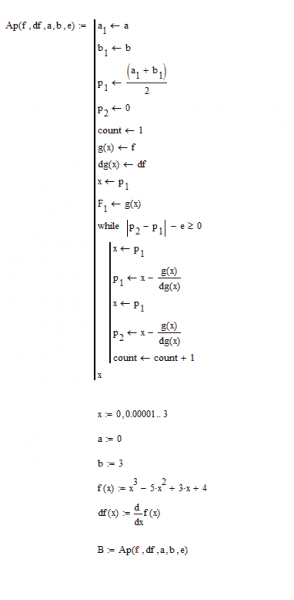• sandy.bridge

## Homework Statement

I am writing a simple program in Mathcad for Newton's Method. The function is x^3-5*x^2+3*x+4. The root is between 0 and 3, and we want a tolerance of 0.00001. My code is the exact same as a program I made in Matlab that works, but since I am new to Mathcad, I am getting an error that I do not know how to fix.

The error I receive is "This value must be a scalar"; it is referring to my function f(x). Here is the code:I don't see why you're making the assignments g(x) ← f and dg(x) ← df. I figure that this is where Mathcad is tripping up because it can't tell at the outset what f is and decides that it's a scalar.

If you get rid of those two assignments and just use f(x) and df(x) inside your function you should be fine.

•sandy.bridge
That certainly fixed it! It definitely was redundant and unnecessary. Thanks!

One other question: do you know a way of implementing Boolean OR in Mathcad? I want to remain in a while loop if any of 3 statements are true. This is rather easy in Matlab, but I am finding it difficult in Mathcad. I am uncertain as to why Mathcad did not include this.

Open the "< ≠ ≥" menu by clicking the icon with the same designation (I have Mathcad 11.2, but presumably the same feature exists in whatever version you are using). You'll find boolean operators there.

Ah, I didn't realize that the V represented OR. Thanks a bunch!